# Initial Situation and Goal

In many cases, measurement curves are provided in groups. Data for the drop in concentration in a liquid over time grouped by different materials are shown in the following graph, for example.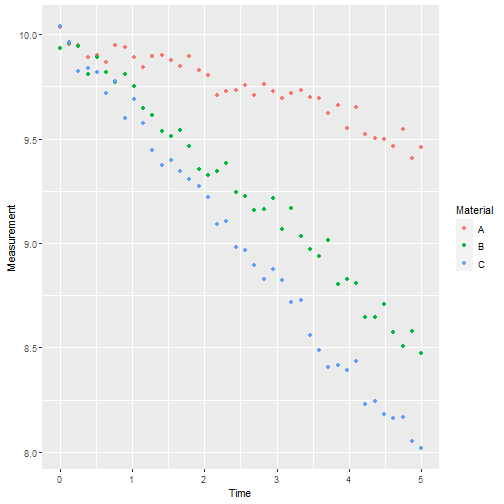A function, in this case a linear function, is to be fitted to each of these curves. The result is shown in the next diagram.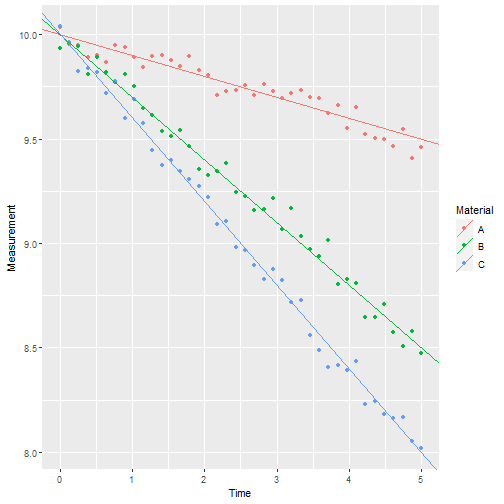If possible, the fits should be made in one step and calculate quality measures for each group.

How do we realize this result in 'Cornerstone' using 'Fit Function' from 'CornerstoneR'?

# Fit Preselected Function

In this example, we will start with the 'Cornerstone' sample data set 'run01' to 'run26'. The data contains 26 different voltage measurements over time, each in a separate file. After we have read all files into a single dataset (see Fundamentals.pdf chapter 7.2.1.3 and 7.2.3) a scatterplot shows the following graph.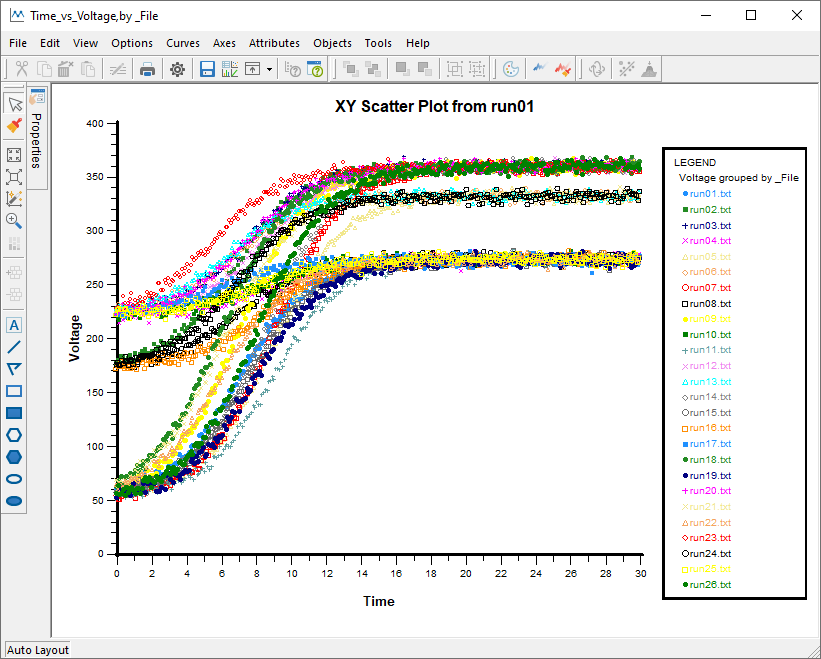We open the corresponding dataset in 'Cornerstone' and choose the menu 'Analysis' -> 'CornerstoneR' -> 'Fit Function via Nonlinear Regression' as shown in the following screenshot.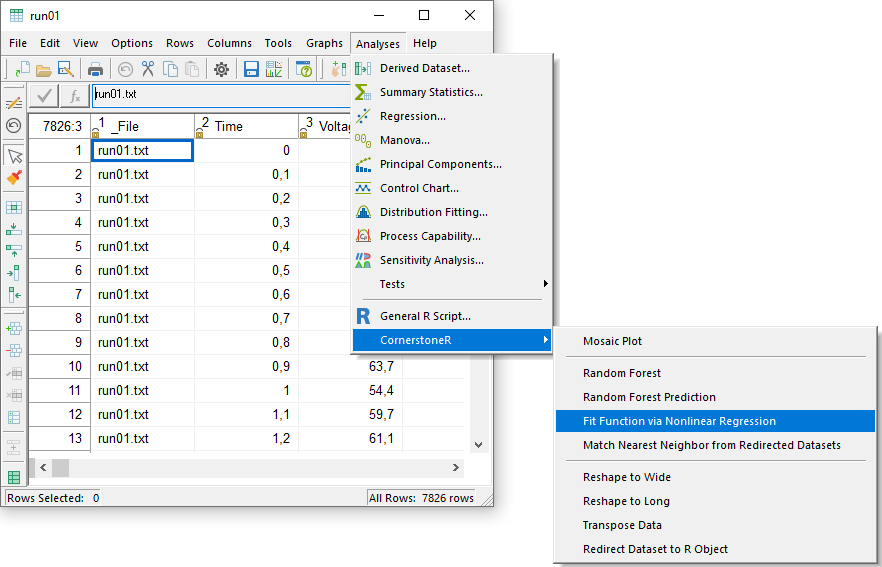In the appearing dialog select variable 'Time' to predictors. 'Voltage' is the response variable. We want a fit for each group in the variable '_File' and select it as group by.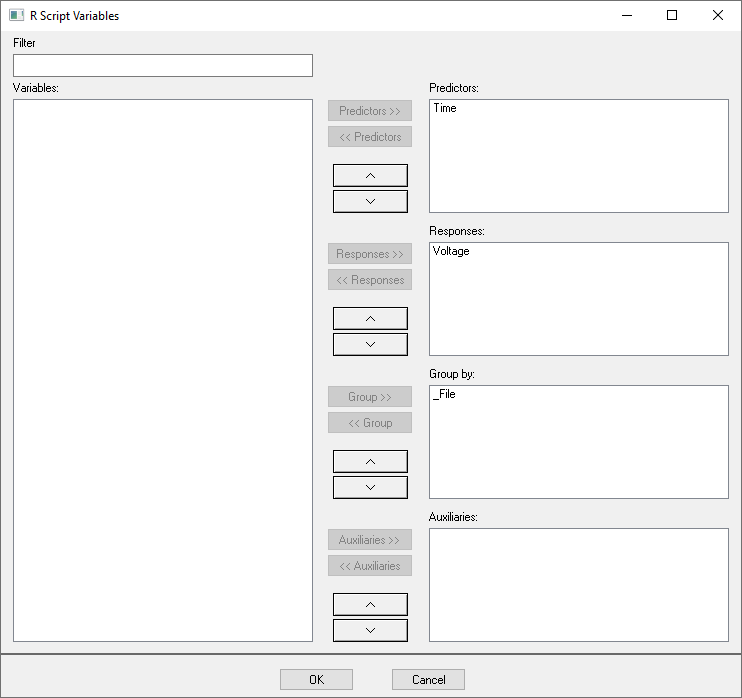'OK' confirms your selection and the following window appears.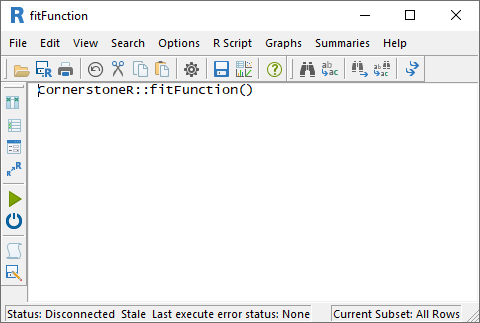Before we start the script it is necessary to select the function we want to fit. To do this we open the menu 'R Script' -> 'Script Variables'.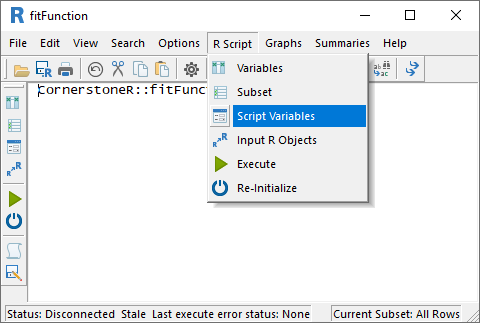In the appearing dialog we select the logistic function 'Logistic' instead of 'User Defined'.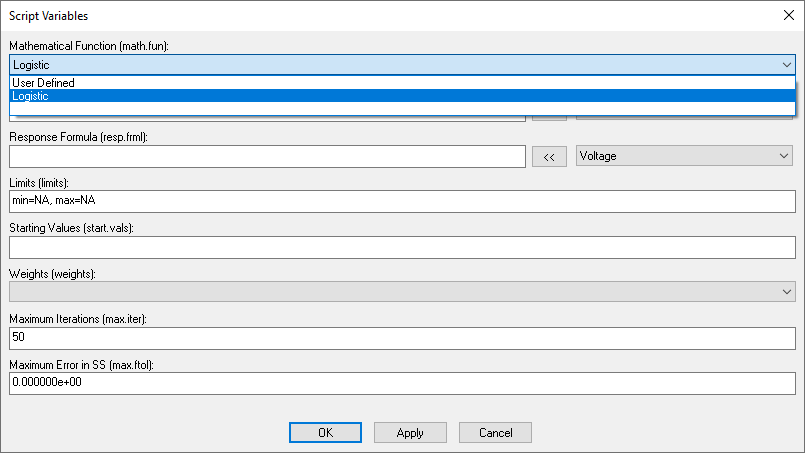A selected function other than 'User defined' gets its settings like predictor and response from the variable selection at the start. Additional settings like starting values use integrated calculations. Limits and Weights are topics in the 'Special Situations' chapter. 'Maximum Iterations' and 'Maximum Error in SS' are stop criteria and can be adjusted if needed.

Now close this dialog via 'OK' and clicking the execute button (green arrow) or choose the menu 'R Script' -> 'Execute' and all calculations are done via 'R'. Calculations are done if the text at the lower left status bar contains 'Last execute error state: OK'. Our result is available via the menus 'Summaries' as shown in the following screenshot.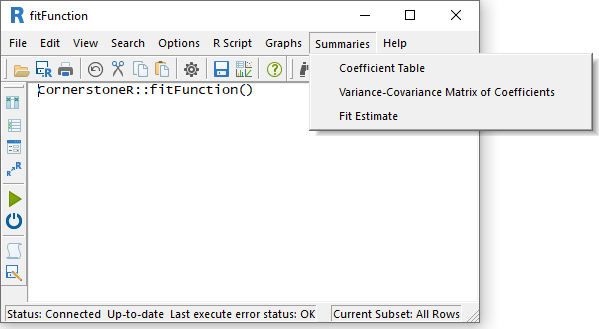The menu 'Fit Estimate' opens a dataset with the group ('_File'), the original response ('Voltage'), the fitted value ('Fitted'), and the corresponding residuals ('Residuals'). To create a graph with the function fit we have to add the predictor ('Time') from the parent dataset via the menu 'Columns' -> 'Add' -> 'From Parent'. A line graph with 'Time' as predictor, 'Fitted' as response, and '_File' as grouping variable results in the following graph.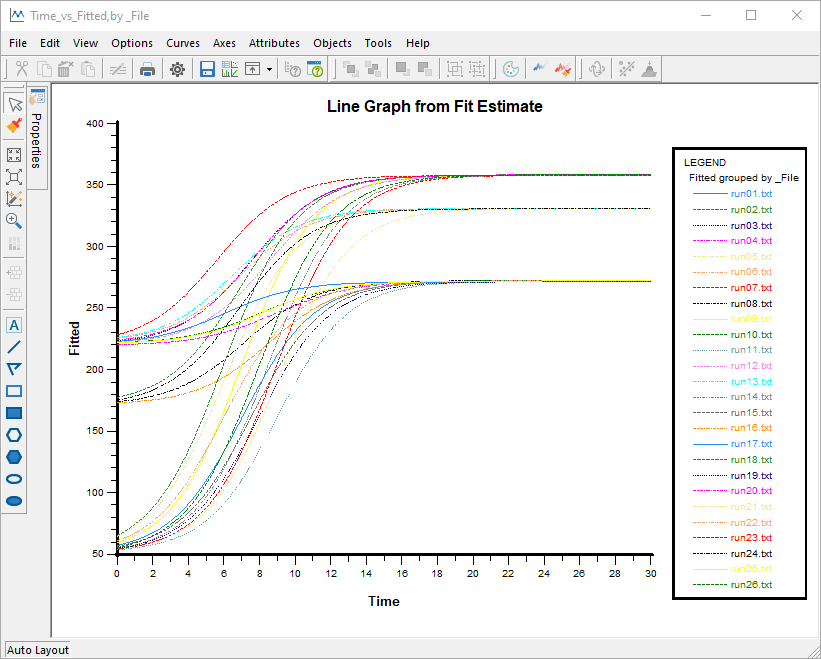To visualize the start end end of each logistic function we can also use a scatter plot of the coefficients 'a' and 'b' as shown in the following graph.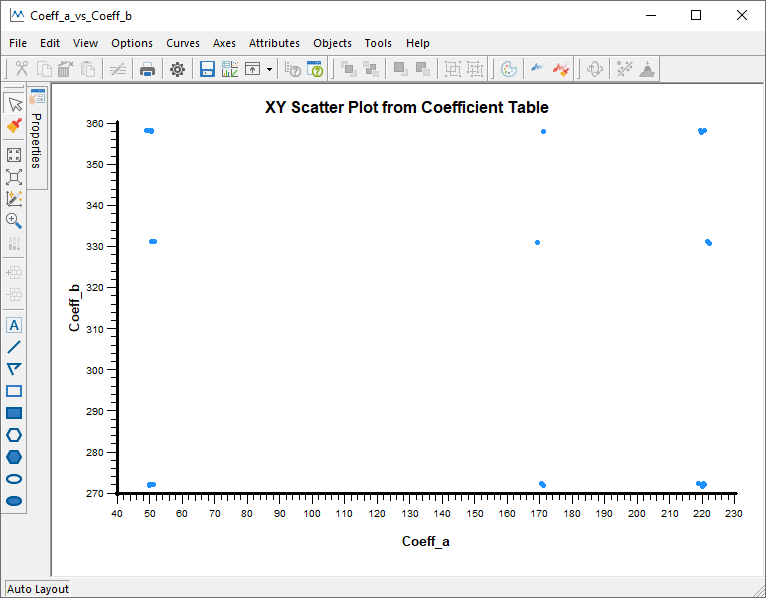# Fit User Defined Function

For this example we open the 'Dissolution' sample dataset in 'Cornerstone' from the 'Regression' subdirectory. This dataset is in wide format and we converted to long format using reshape grouped data to long. As a result we get a dataset like in the following screenshot. I renamed the columns 'variable1' and 'variable2' to 'group1' and 'group2' for a better identification.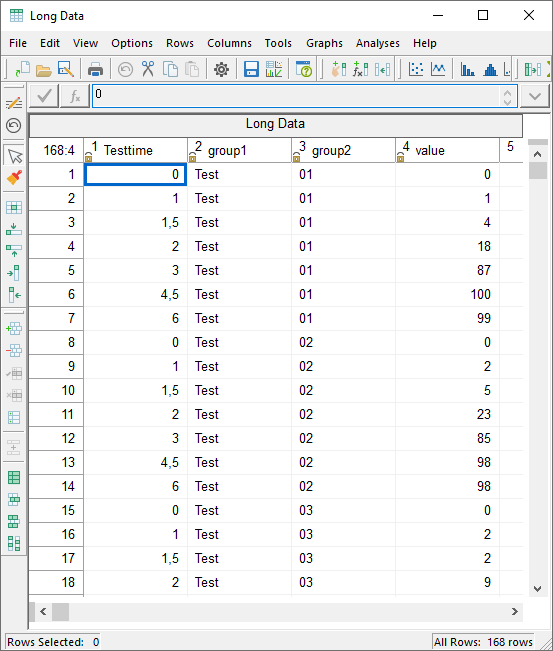From this dataset in 'Cornerstone' we start the to fit a function like in the first example via the menu 'Analysis' -> 'CornerstoneR' -> 'Fit Function via Nonlinear Regression'. In the appearing dialog we select 'Testtime' as predictor, 'value' as response, and 'group1' and 'group2' as grouping variables like in the following screenshot.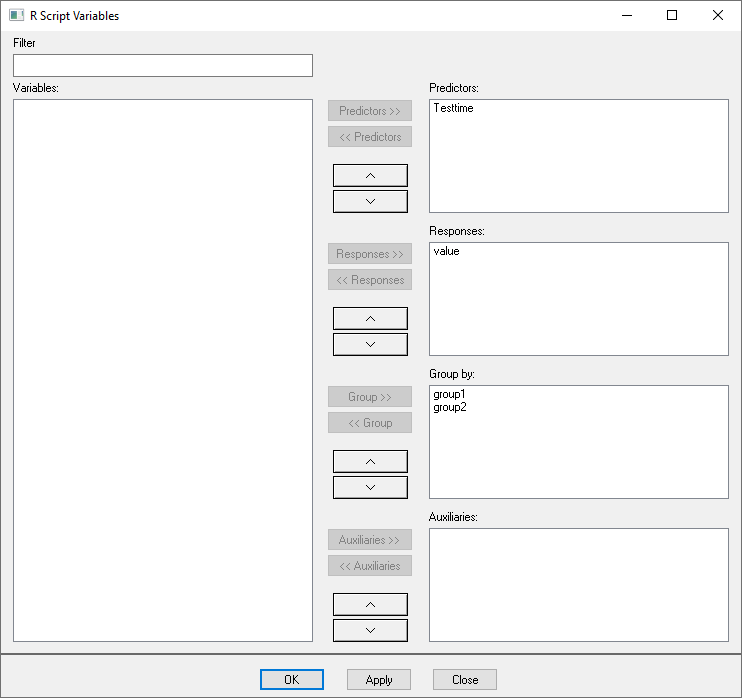After 'OK' the known 'R Script' dialog appears. Here we select the menu 'R Script' -> 'Script Variables' and use now the default setting 'User Defined' to fit a Weibull model to the Dissolution data.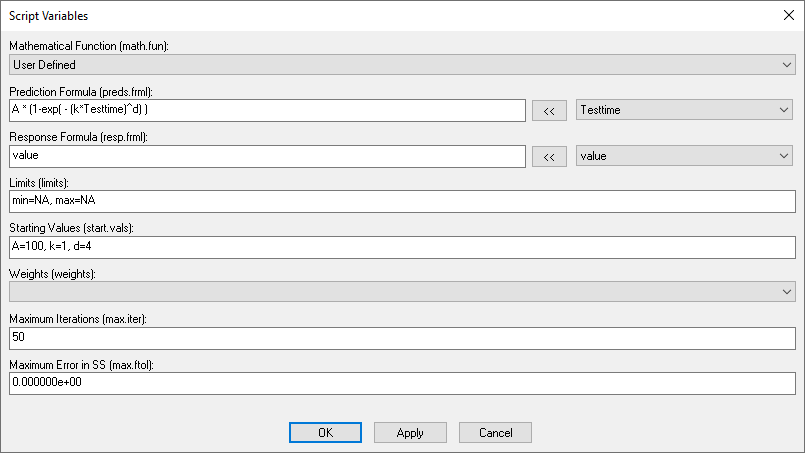The influence and target formula together form an equation of the form f(y)=g(x). In our case we set the prediction formula (right-hand side g(x)) to the formula of the Weibull model as shown in the screenshot. The response formula (left-hand side f(y)) is solely our response. For both text boxes we can use the button '<<' and the drop-down box arranged on the left to add variables in a simple way. We can also add a function to the response formula like 'log(value)' if it improves the fit. As last step we set start values for each variable.

Now we close this dialog via 'OK' and execute the script (green arrow) by the menu 'R Script' -> 'Execute'. One result is the dataset with all coefficients for the 24 groups as shown in the following screenshot.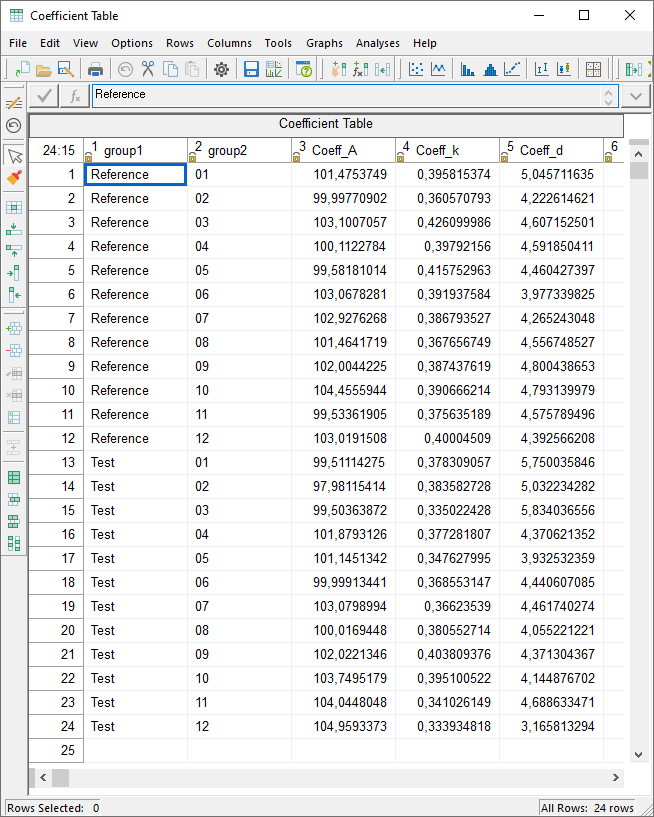From this dataset it is possible, for example, to create a multi-vari chart of the first coefficient, as shown in the following graph.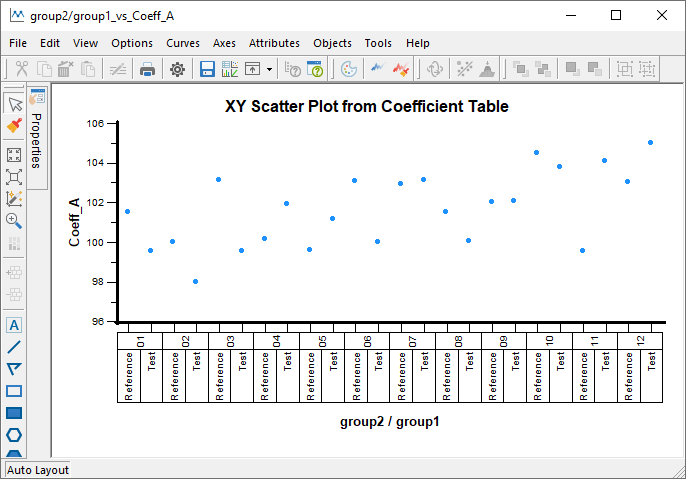# Special Situations

This chapter briefly discusses special situations which can be handled via 'fitFunction'.

## Limits: Flattened Sinusoidal Oscillation

Assume a situation where your data looks like the curve in the following graph.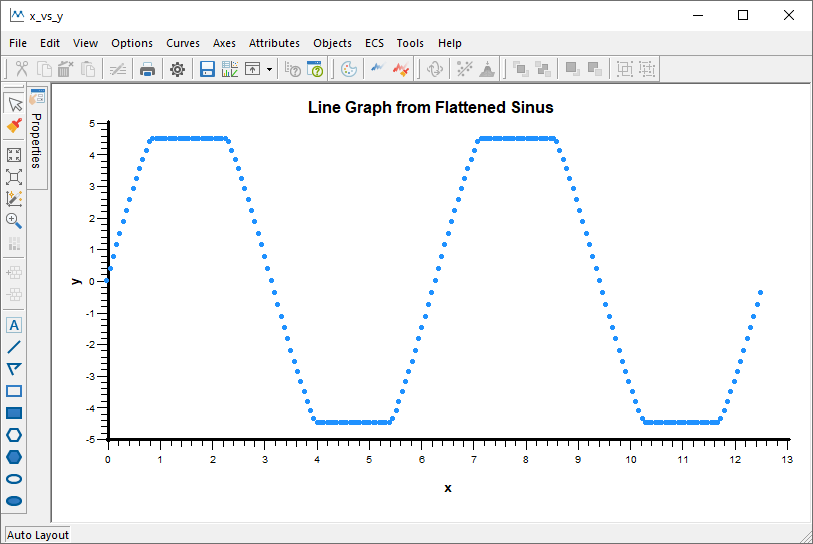Obviously the data is based on a sinusoidal oscillation which was censored, e.g., by a measuring instrument. The poor fit of a sinusoidal function is shown in the next graph.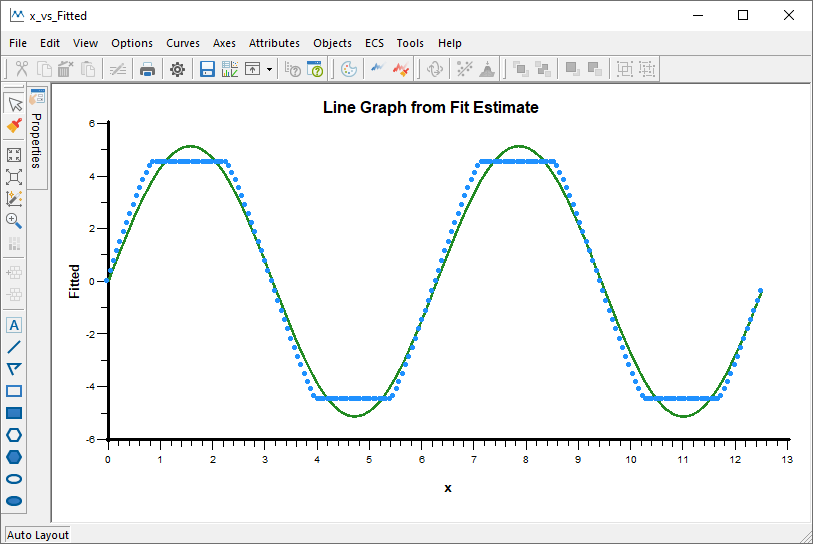Limits reflect this behavior and can be handled like an additional coefficient. The following screenshot shows the use of a coefficient 'c' for the 'min' and 'max' limit.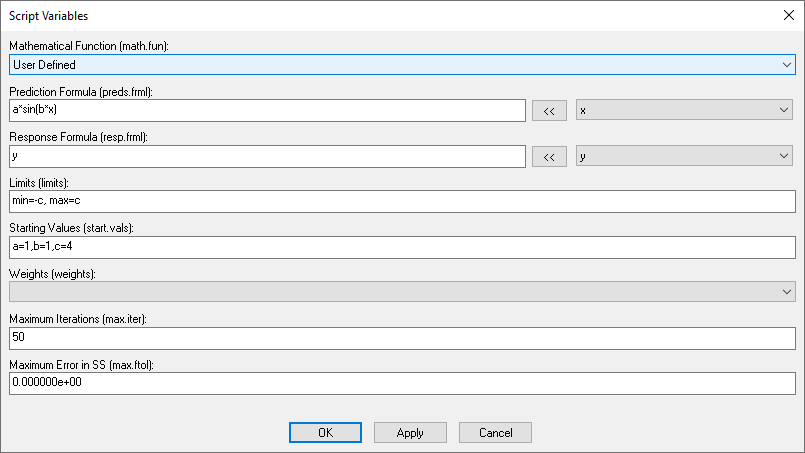The resulting fit is shown in the final graph.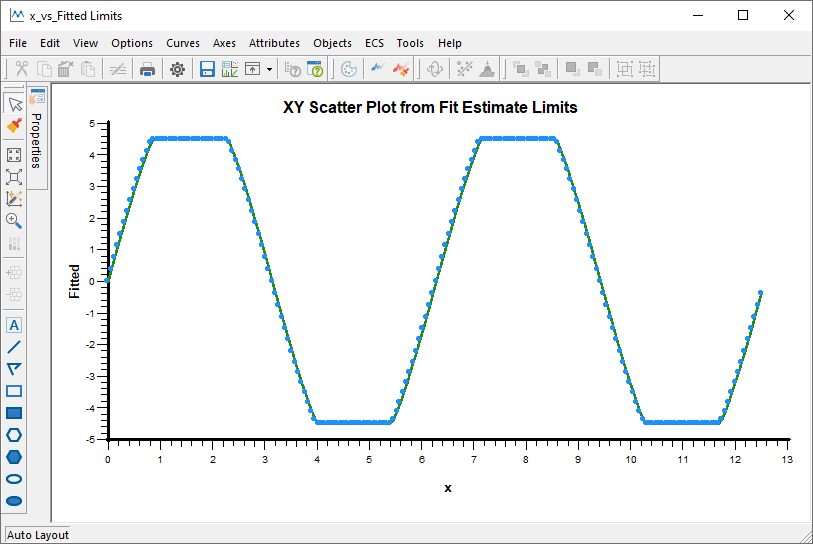## Weights: Small Example

Fitting a linear function (green) to data in a hyperbolic form (blue) results in the following graph.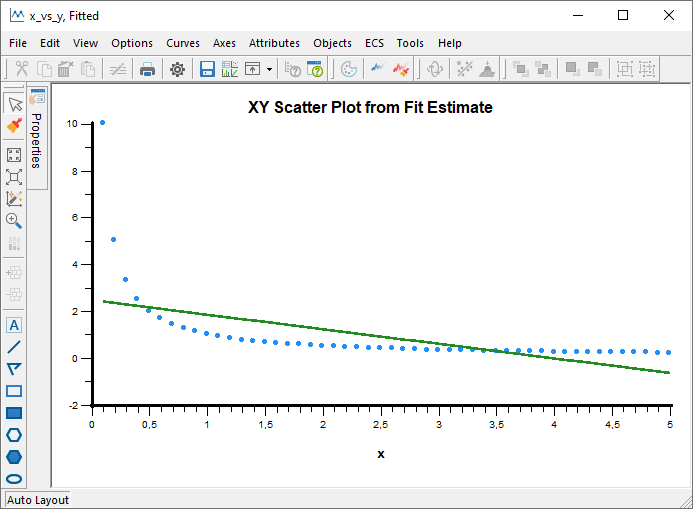Generalizing the nonlinear least square algorithm to a weighted fit is done when you select a 'Weight' variable in the 'Script Variables' dialog as shown in the following screenshot.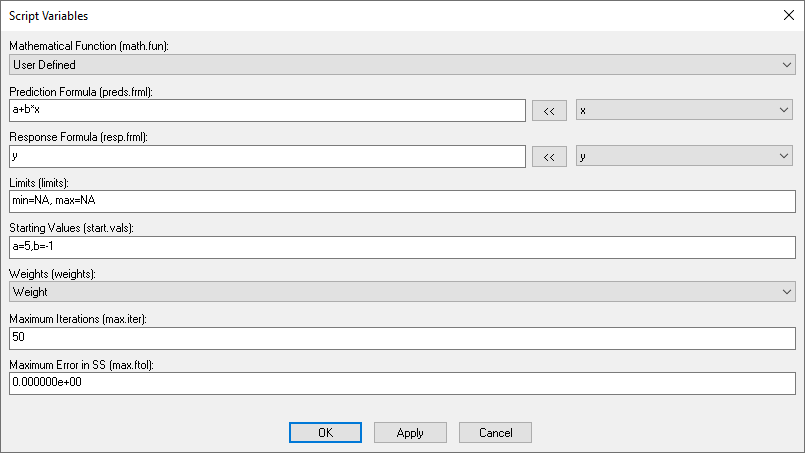The underlying variable contains a high weighting of data in the margin and a low weighting of data in the middle. This results in the following graph.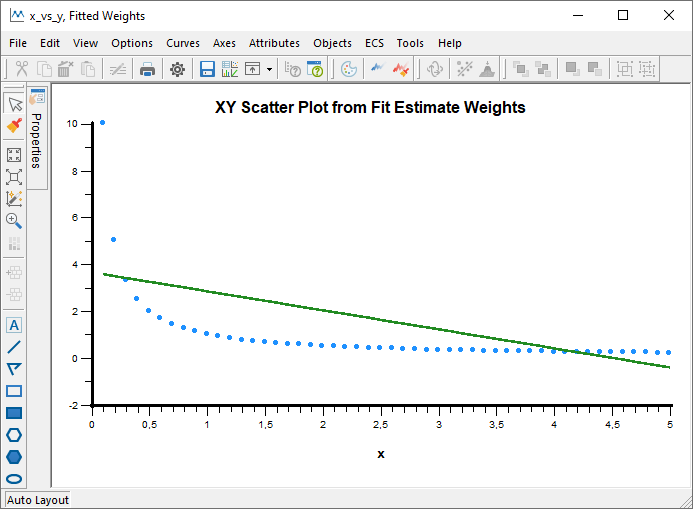The line is shifted accordingly by the weighting in the margin.